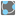• # Is there an error in the checkio() example shown?

## Question related to missionThree Points Circle

I think there is an error in the example given for the 'Three Points Circle' problem. In the first graph the points 2,2 2,6 6,2 are shown with the output returning a center at 4,4 and a radius or 2.83. In the example below showing the checkio() input/output the points 2,2 4,2 2,2 are input with the same output as the graph example. Using that input I,m getting a center at 3,3 and radius of 1.41.

I'm using the perpendicular bisector method (there MUST be an easier way) but not quite ready for prime time.

Thanks.8